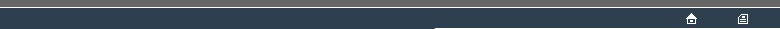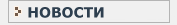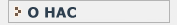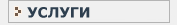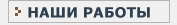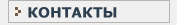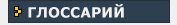СТАТИСТИЧЕСКИЙ В данном разделе Вы найдете все интересующие Вас термины и определения, связанные с статистикой и статистическими исследованиями.КЛИНИЧЕСКИЕ ИССЛЕДОВАНИЯ Необходимый перечень терминов и определений, используемых при проведении клинических испытанийM MANN-WHITNEY TEST [Devised by ()] This is a test of difference in location for an EXPERIMENTAL DESIGN involving two samples with data measured on an ORDINAL SCALE or better. The TEST STATISTIC is a measure of ordinal precedence. For each possible pairing of an observation in one group with an observation in the alternate group, the pair is classified in one of three ways - according to whether the difference is positive, zero or negative; the numbers in these three categories are tallied over the RANDOMISATION SET. The RANDOMISATION SET is the same as that for the PITMAN PERMUTATION TEST(1). This test is generally recommended for comparisons involving ORDINAL-SCALE data but is not confined to this SCALE-TYPE. An equivalent formulation of the test, based upon ranking the data and summing ranks within groups, is the WILCOXON TEST(2). Also see : COMBINATIONS. MEASUREMENT TYPE This is a distinction regarding the relationship between a phenomenon being measured and the data as recorded. The main distinctions are concerned with the meaningfulness of numerical comparisons of data (NOMINAL SCALE versus ORDINAL SCALE versus INTERVAL SCALE versus RATIO SCALE : this is known as STEVENS' TYPOLOGY), whether the scale of the measurements (other than NOMIMAL SCALE measurements) should be regarded as essentially conituous or discrete, and whether the scale is bounded or unbounded. MID-P [Proposed by H.O Lancaster(), and further promoted by G.A. Barnard] This is a TAIL DEFINITION POLICY that the ALPHA value should be calculated as the sum of the proportion of the TAIL for data strictly more extreme than the OUTCOME, plus one half of the proportion of the DISTRIBUTION corresponding to the exact OUTCOME value. This gives an unbiased estimate of ALPHA. MINIMAL-CHANGE SEQUENCE Exploration of a RANDOMISATION DISTRIBUTION is such a sequence that the successive RANDOMISATION(3)s differ is a simple way. In the context of a RANODMISATION TEST this can mean that the value of the TEST STATISTIC for a particular RANDOMISATION(3) may be calculated by a simple adjustment to the value for the preceding RANDOMISATION(3). Also see : RANDOMISATION(1). MONTE-CARLO TEST [Named after the famous site of gambling casinos] A MONTE-CARLO TEST involves generating a random subset of the RANDOMISATION SET, sampled without replacement, and using the values of the TEST STATISTIC to generate an estimate of the form of the full RANDOMISATION DISTRIBUTION. This procedure is in contrast to the BOOTSTRAP procedure in that the sampling of the RANDOMISATION SET is without replacement. An advantage of the MONTE-CARLO TEST over the BOOTSTRAP is that with successive resamplings it converges to the GOLD STANDARD(1) form of the EXACT TEST(1). An effective necessity for the MONTE-CARLO procedure is a source of random codes or an effective PSEUDO-RANDOM generator. MULTINOMIAL DISTRIBUTION This is the distribution of outcomes expected if a certain number of independent trials are undertaken of a several separate BERNOUILLI PROCESSes, to determine a number of alternative outcomes. A special case, where the number of outcomes is 2, is the BINOMIAL DISTRIBUTION. The distribution depends upon the collection of parameter values of the corresponding BERNOULLI PROCESSes and upon the number of trials, 'n'. An alternative characterisation is as the outcome of a number of separate POISSON PROCESSes with separate rate parameters. Also see : TWO-WAY TABLEs.M
MANN-WHITNEY TEST
MEASUREMENT TYPE
MID-P
MINIMAL-CHANGE SEQUENCE
MONTE-CARLO TEST
MULTINOMIAL DISTRIBUTIONНовости   О нас   Услуги   Наши работы   Статьи   Контакты   Глоссарий
Статистическая помощь! © 2005 - 2023 • Защита авторских прав